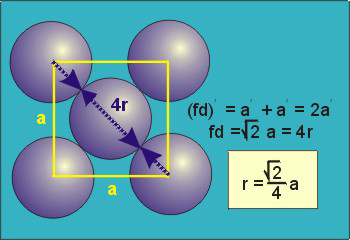# What is the density of a metal that has atomic mass 114 u and crystallizes in a face-centred cubic cell with an edge length of 105 pm?

May 17, 2017

The density is ${\text{28.9 g/cm}}^{3}$.

Calculate the mass of the unit cellAn fcc unit cell contains

8 color(red)(cancel(color(black)("corners"))) × (1/8 "atom")/(1 color(red)(cancel(color(black)("corner")))) + 6 color(red)(cancel(color(black)("faces"))) × (1/2 "atom")/(1 color(red)(cancel(color(black)("face")))) = "1 atom + 3 atoms = 4 atoms"

$\text{Mass" = 4 color(red)(cancel(color(black)("atoms"))) × (1 color(red)(cancel(color(black)("mol"))))/(6.022 × 10^23 color(red)(cancel(color(black)("atoms")))) × "114 g"/(1 color(red)(cancel(color(black)("mol")))) = 7.57 ×10^"-22"color(white)(l) "g}$

Calculate the volume of the unit cellThe diagonal along a face is $d = 4 r$.

${d}^{2} = {a}^{2} + {a}^{2} = 2 {a}^{2} = {\left(4 r\right)}^{2} = 16 {r}^{2}$

${a}^{2} = 8 {r}^{2}$

a = rsqrt8 = sqrt8 × 105 × 10^"-12" color(red)(cancel(color(black)("m"))) × "100 cm"/(1 color(red)(cancel(color(black)("m")))) = 2.97 × 10^"-8" color(white)(l)"cm"

V = a^3 = (2.97 × 10^"-8" color(white)(l)"cm")^3 = 2.62 × 10^"-23"color(white)(l) "cm"^3

Calculate the density

ρ = m/V = (7.57 ×10^"-22"color(white)(l) "g")/(2.62 × 10^"-23"color(white)(l) "cm"^3) = "28.9 g/cm"^3